EXERCISE 7.7

1). Represent the following on the number line.
i)  2/6,  ii)  4/6, iii)  7/6 ,  iv)  10/6
Ans.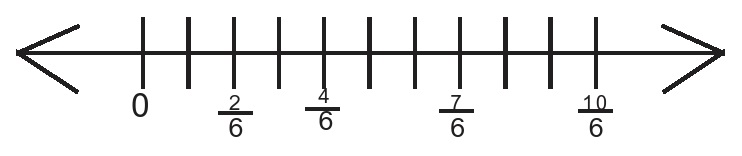2). Write the fraction representing the shaded portion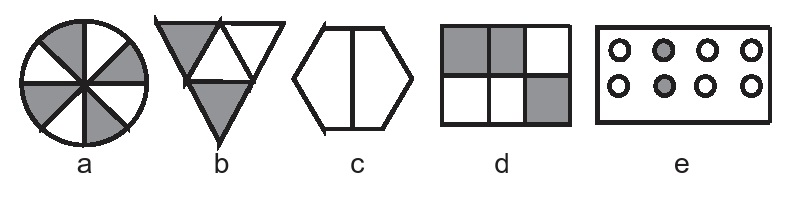Ans .         a)  4/8                    b)  2/4                  c)  no region                        d)  3/6                                  e)  2/8

3). Identify proper and improper fraction
6/9 ,   7/12 ,   4/8 ,   10/5 ,   25/4 ,   2/9 ,   18/12 ,   15/5 ,   3/2 ,   3/7
Ans.
Proper fraction = 6/9 ,  7/12 ,  4/8 ,  2/9 ,  3/7
Improper fraction = 10/5 ,  25/4 ,  18/12 ,  15/5 ,   3/2

4). Identify the unlike fraction from the following
7/11 ,  1/2 ,  4/11 ,  5/7 , 9/12,  15/11,  2/11
Ans. Unlike fractions = 1/2 ,  5/7 ,  9/12.

5). Identify like fraction from the following

13/5 ,  9/12 ,  1/2 , 3/9 ,  7/5 ,  10/5 ,  3/4 , 18/5 ,  7/10
Ans. Like fractions = 13/5 ,  7/5 , 10/5 ,  18/5

6). Convert the following improper fraction into like fraction.
a)  13/2         b)  28/5       c) 45/6        d) 38/(9 )
Ans.
a)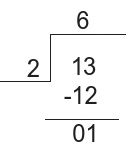∴   13/2  =  6 ½

b)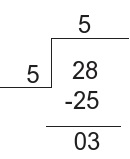∴  28/5  =  5 ³⁄ 5
c)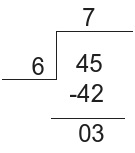∴  45/6  =  7½
d)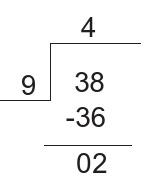∴   38/9 = 4²⁄ 9

7). Write the following fraction into ascending and descending orders using {< , >}
5/12 ,  8/12 ,  1/12 ,  4/12 ,  11/12 ,  0/12 ,  7/12
Ans. Ascending order = 0/12 < 1/12 < 4/12 < 5/12 < 7/12 < 8/12 < 11/12
Descending order = 11/12 > 8/12 > 7/12 > 5/12 > 4/12 > 1/12 > 0/12

8). Color the parts according to the fraction given.
a) 3/8      b) 3/4        c) 6/8        d) 5/89). Write.
a) Any 2 proper fraction and 2 improper fractions with denominator 5
b) Any 3 proper fraction and 3 improper fractions with denominator 11
Ans.
a) Proper fractions :- 2/5  and  3/5

Improper fractions :- 7/5  and  9/5
b) Proper fractions :- 7/11  and  9/11
Improper fractions :- 15/11  and  19/11

10). Compare the following fractions
1) 3/4 And 2/5             2) 4/5 And 5/6          3) 32/4 And 21/4        4) 7/11 And 2/11          5) 31/3 And 32/6
Ans.
1) 3/4 and 2/5.            Here
LCM = 20

=  (3×5)/(4×5) = 15/20 , (2×4)/(5×4) = 8/20
=  15/20 > 8/20
=  3/4 > 2/5

2) 4/5 and 5/6.              Here LCM = 30
= (4×6)/(5×6) = 24/30 , (5×5)/(6×5) = 25/30
= 24/30 < 25/30
= 4/5 < 5/6

3) 32/4 and 21/4
= (3×4+2)/4 = 14/4 , (2×4+1)/4 = 9/4
= 14/4 > 9/4

= 32/4 > 21/4

4) 7/11 and 2/11
= 7/11 > 2/11

5) 31/3 and 32/6.           Here LCM =6
= (3×3+1)/3 = 10/3 ,   (3×6+2)/6 = 20/6
= (10 ×2)/(3×2) = 20/6 ,    (20×1)/(6×1) = 20/6
= 20/6 = 20/6
= 31/3 = 32/6

11) Reduce the following fractions to their simplest form
a) 16/72      b) 80/24       c) 15/75      d) 42/28
Ans:
a) 16/72
HCF of 16 and 72 is 8

= (16÷8) / (72÷8) =  2/9
b) 80/24                                              HCF of 80 and 24 is 8
= (80÷8)/(24÷8) = 10/3
c) 15/75                                               HCF of 15 and 75 is 15
= (15÷15)/(75÷15) = 1/5
d) 42/28                                            HCF of 42 and 28 is 14
= (42÷14)/(28÷14) = 3/2½

12). Meenakshi ate 2½ Chapattis for breakfast and 1 Chapatti for lunch. How many chapatti did she eat?

Ans. Fraction of chapattis eaten for breakfast = 2½
Fraction of chapatti eaten for lunch = 1½
Total chapattis eaten by Meenakshi
= 2½ + 1½
= 5/2 + 3/2
= 8/2 = 4
Therefore Meenakshi ate a total of 4 chapattis.

13). Joseph cleaned 2/3 of his room. His sister Tina helped him clean more 1/5 of his room. How much did they clean together? What part of the whole space is left to be cleaned?

Ans. Fraction of place cleaned by Joseph = 2/3
Total space cleaned by both = 2/3 + 1/3
= (2×5)/(3×5) + (1×3)/(5×3)
= (10+3)/15 = 13/15
Space left to be cleaned is 1−13/15
= (1×15)/(1×15) – (13×1)/(15×1)
= 15/15 − 13/15
= (15−13)/15 = 2/15
Hence both of them cleaned 13/15 of Joseph’s room and 2/15 is remaining to be cleaned